Optics Exam2 and Problem Solutions

Optics Exam2 and  Problem Solutions

1. Look at the given picture below. Two concave mirrors are placed on same principal axis. Find focal points of mirror 2 in terms of d.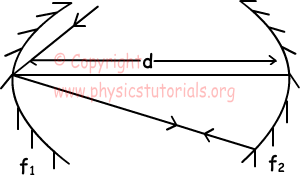Ray hits the vertex ofmirror 1 and reflects with same angle. Ray, coming from first mirror turns back with same path after reflecting from second mirror. Thus, we infer that, first mirror is placed at the center of second mirror. So focal point of the second mirror is;
d=2f2

f2=d/2

2. Find the relation between the focal points of the concave mirrors given below.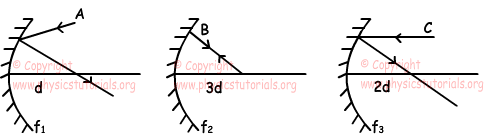Since extension of the ray A passes from behind of the mirror, f1 is bigger than d. In the second picture, ray B turns back on itself. Thus; 3d is the center of the mirror and f2=3d/2=1,5d. In third picture, ray coming parallel to principal axis passed from focal point of the mirror. So; f3=2d.

3. Find shape of image of the object given below.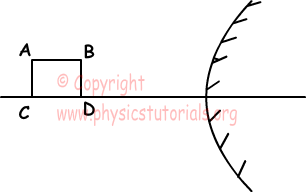Ray coming from AB parallel to the principle axis reflects from convex mirror and it goes as if it comes from focal point of the mirror. Thus, image of the points A, B are on the extension of reflected ray. AC side of the image is shorter than the BD side since distance between AC and mirror is larger than the distance between BD and mirror. Image of the object is shown in the picture given below;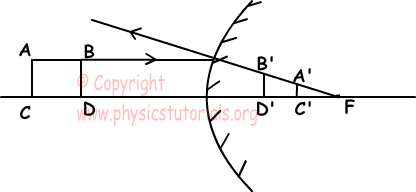4. Focal point of the concave mirror given below is 120 cm. An object having length 8 cm is placed at 60 cm away from the mirror. Find the location and height of the image.

1/f=1/dobject+1/dimage

1/120=1/60+1/dimage

dimage=-120cm

Hobject/Himage=dobject/dimage

8/Himage=60/120

Himage=16 cm

5. Concave mirror and convex mirror having equal focal lengths 2f are placed on same principal axis. If the object AB is placed between these mirrors, find the height ratio of the images of this object on two mirrors Hx/Hy=?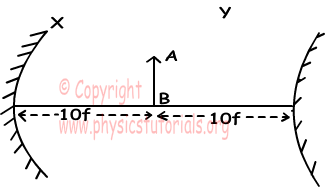We use following equation to find height of the object;

Himage/Hobject=f/df  where df is the distance between object and focal point of the mirror.

Distance between object and focal point of the mirror x is dfx=8f and distance between object and focal point of the mirror y is dfy=12f.

Let height of the object is h;

HimageX/h=2f/8f

HimageX=h/4

HimageY/h=2f/12f

HimageY=h/6

HimageX/HimageY=3/2

Author: